# Side by side charts in SPSS

One aspect of SPSS charts that you need to use syntax for is to create side-by-side charts. Here I will illustrate a frequent use case, time series charts with different Y axes. You can download the data and code to follow along here. This is data for Buffalo, NY on reported crimes from the UCR data tool.

So after you have downloaded the csv file with the UCR crime rates in Buffalo and have imported the data into SPSS, you can make a graph of violent crime rates over time.

``````*Making a chart of the violent crime rate.
GGRAPH
/GRAPHDATASET NAME="graphdataset" VARIABLES=Year ViolentCrimerate MISSING=LISTWISE
REPORTMISSING=NO
/GRAPHSPEC SOURCE=INLINE.
BEGIN GPL
SOURCE: s=userSource(id("graphdataset"))
DATA: Year=col(source(s), name("Year"))
DATA: ViolentCrimerate=col(source(s), name("ViolentCrimerate"))
GUIDE: axis(dim(1), label("Year"))
GUIDE: axis(dim(2), label("Violent Crime Rate per 100,000"))
ELEMENT: line(position(Year*ViolentCrimerate))
ELEMENT: point(position(Year*ViolentCrimerate), color.interior(color.black), color.exterior(color.white), size(size."7"))
END GPL.``````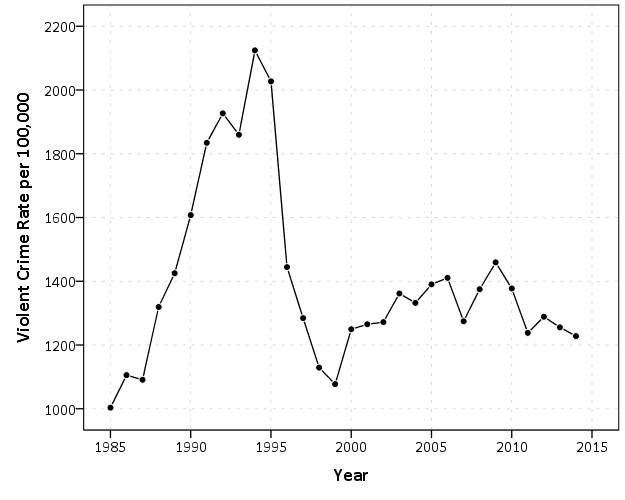I like to superimpose the points on simple line charts, to reinforce where the year observations are. Here we can see that there is a big drop post 1995 for the following four years (something that would be hard to say exactly without the points). Part of the story of Buffalo though is the general decline in population (similar to most of the rust belt part of the nation since the 70’s).

``````*Make a chart of the population decline.
GGRAPH
/GRAPHDATASET NAME="graphdataset" VARIABLES=Year Population MISSING=LISTWISE
REPORTMISSING=NO
/GRAPHSPEC SOURCE=INLINE.
BEGIN GPL
SOURCE: s=userSource(id("graphdataset"))
DATA: Year=col(source(s), name("Year"))
DATA: Population=col(source(s), name("Population"))
GUIDE: axis(dim(1), label("Year"))
GUIDE: axis(dim(2), label("Population"))
ELEMENT: line(position(Year*Population))
ELEMENT: point(position(Year*Population), color.interior(color.black), color.exterior(color.white), size(size."7"))
END GPL.``````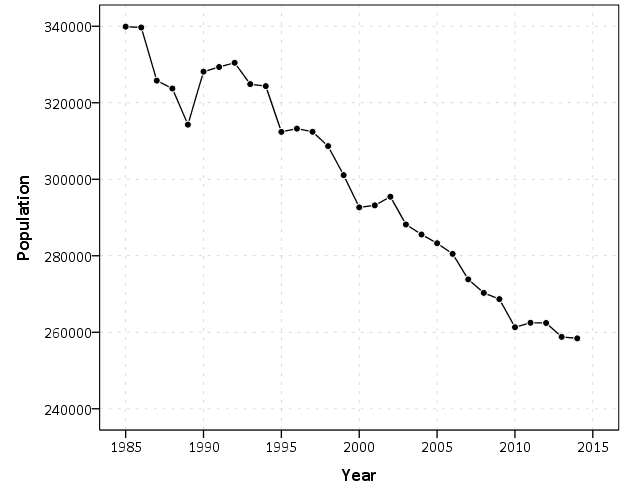Now we want to place these two charts over top of one another. So check out the syntax below, in particular to `GRAPH: begin` statements.

``````*Now put the two together.
GGRAPH
/GRAPHDATASET NAME="graphdataset" VARIABLES=Year Population ViolentCrimerate
/GRAPHSPEC SOURCE=INLINE.
BEGIN GPL
SOURCE: s=userSource(id("graphdataset"))
DATA: Year=col(source(s), name("Year"))
DATA: Population=col(source(s), name("Population"))
DATA: ViolentCrimerate=col(source(s), name("ViolentCrimerate"))
GRAPH: begin(origin(14%,12%), scale(85%, 60%))
GUIDE: axis(dim(1), label("Year"), opposite())
GUIDE: axis(dim(2), label("Violent Crime Rate per 100,000"))
ELEMENT: line(position(Year*ViolentCrimerate))
ELEMENT: point(position(Year*ViolentCrimerate), color.interior(color.black), color.exterior(color.white), size(size."7"))
GRAPH: end()
GRAPH: begin(origin(14%, 75%), scale(85%, 20%))
GUIDE: axis(dim(1), label("Year"))
GUIDE: axis(dim(2), label("Population"))
ELEMENT: line(position(Year*Population))
ELEMENT: point(position(Year*Population), color.interior(color.black), color.exterior(color.white), size(size."7"))
GRAPH: end()
END GPL.    ``````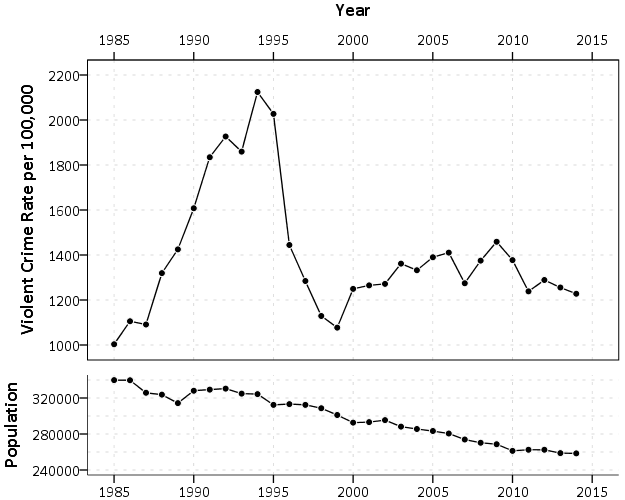In a nutshell, the graph begin statements allow you to chunk up the graph space to make different/arbitrary plots. The percentages start in the top right, so for the first violent crime rate graph, the origin is listed as 14% and 12%. This means the graph starts 14% to the right in the overall chart space, and 12% down. These paddings are needed to make room for the axis labels. Then for the scale part, it lists it as 85% and 60%. The 85% means take up 85% of the X range in the chart, but only 60% of the Y range in the chart. So this shows how to make the violent crime chart take a bigger proportion. of the overall chart space than the population chart. You can technically do charts with varying axes in SPSS without this, but you would have to make the panels take up an equal amount of space. This way you can make the panels whatever proportion you want.

For Buffalo the big drop in 1996 is largely due to a very large reduction in aggravated assaults (from over 3,000 in 1995 to under 1,600 in 1996). So here I superimpose a bar to viz. the proportion of all violent crimes. This wouldn’t be my first choice of how to show this, but I think it is a good illustration of how to superimpose and/or stack additional charts using this same technique in SPSS.

``````*Also superimposing a stacked bar chart on the total types of crimes in the background.
COMPUTE PercentAssault = (Aggravatedassault/ViolentCrimeTotal)*100.
FORMATS PercentAssault (F2.0).
EXECUTE.

GGRAPH
/GRAPHDATASET NAME="graphdataset" VARIABLES=Year Population ViolentCrimerate PercentAssault
/GRAPHSPEC SOURCE=INLINE.
BEGIN GPL
SOURCE: s=userSource(id("graphdataset"))
DATA: Year=col(source(s), name("Year"))
DATA: Population=col(source(s), name("Population"))
DATA: ViolentCrimerate=col(source(s), name("ViolentCrimerate"))
DATA: PercentAssault=col(source(s), name("PercentAssault"))
GRAPH: begin(origin(14%,12%), scale(75%, 60%))
GUIDE: axis(dim(1), label("Year"), opposite())
GUIDE: axis(dim(2), label("Violent Crime Rate per 100,000"))
ELEMENT: line(position(Year*ViolentCrimerate))
ELEMENT: point(position(Year*ViolentCrimerate), color.interior(color.black), color.exterior(color.white), size(size."7"))
GRAPH: end()
GRAPH: begin(origin(14%, 75%), scale(75%, 20%))
GUIDE: axis(dim(1), label("Year"))
GUIDE: axis(dim(2), label("Population"))
ELEMENT: line(position(Year*Population))
ELEMENT: point(position(Year*Population), color.interior(color.black), color.exterior(color.white), size(size."7"))
GRAPH: end()
GRAPH: begin(origin(14%, 12%), scale(75%, 60%))
SCALE: linear(dim(2), min(0), max(60))
GUIDE: axis(dim(1), null())
GUIDE: axis(dim(2), label("Percent Assault"), opposite(), color(color.red), delta(10))
ELEMENT: bar(position(Year*PercentAssault), color.interior(color.red), transparency.interior(transparency."0.7"), transparency.exterior(transparency."1.0"), size(size."5"))
GRAPH: end()
END GPL.``````While doing multiple time series charts is a common use, you can basically use your imagination about what you want to accomplish with this. Another common example is to put border histograms on scatterplot (which the GPL reference guide has an example of). Here is an example I posted recently to Nabble that has the number of individuals at risk in a Kaplan-Meier plot.# Heatmaps in SPSS

Heatmap is a visualization term that gets used in a few different circumstances, but here I mean a regular grid in which you use color to indicate particular values. Here is an example from Nathan Yau via FlowingData: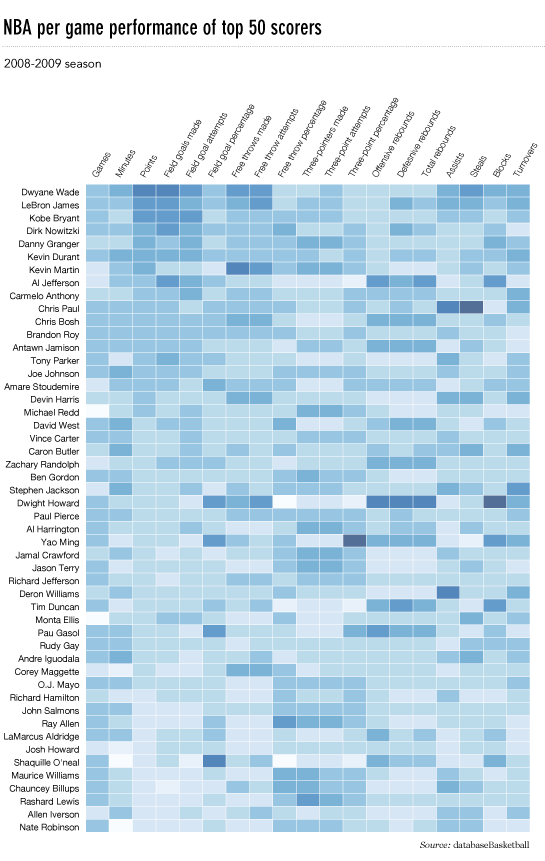They are often not the best visualization to use to evaluate general patterns, but they offer a mix of zooming into specific individuals, as well as to identify overall trends. In particular I like using them to look at missing data patterns in surveys in SPSS, which I will show an example of in this blog post. Here I am going to use a community survey for Dallas in 2016. The original data can be found here, and the original survey questions can be found here. I’ve saved that survey as an SPSS file you can access at this link. (The full code in one sps syntax file is here.)

So first I am going to open up the data file from online, and name the dataset `DallasSurv16`.

``````*Grab the data from online.
SPSSINC GETURI DATA
URI="https://dl.dropbox.com/s/5e07yi9hd0u5opk/Surv2016.sav?dl=0"
FILETYPE=SAV DATASET=DallasSurv16.``````

Here I am going to illustrate making a heatmap with the questions asking about fear of crime and victimization, the Q6 questions. First I am going to make a copy of the original dataset, as we will be making some changes to the data. I do this via the `DATASET COPY` function, and follow it up by activating that new dataset. Then I do a frequency to check out the set of Q6 items.

``````DATASET COPY HeatMap.
DATASET ACTIVATE HeatMap.
FREQ Q6_1Inyourneighborhoodduringthe TO Q69Fromfire.``````

From the survey instrument, the nine Q6 items have values of 1 through 5, and then a "Don’t Know" category labeled as 9. All of the items also have system missing values. First we are going to recode the system missing items to a value of 8, and then we are going to sort the dataset by those questions.

``````RECODE Q6_1Inyourneighborhoodduringthe TO Q69Fromfire (SYSMIS = 8)(ELSE = COPY).
SORT CASES BY Q6_1Inyourneighborhoodduringthe TO Q69Fromfire.``````

You will see the effect of the sorting the cases in a bit for our graph. But the idea about how to make the heatmap in the grammar of graphics is that in your data you have a variable that specifies the X axis, a variable for the Y axis, and then a variable for the color in your heatmap. To get that set up, we need to go from our nine separate Q6 variables to one variable. We do this in SPSS by using `VARSTOCASES` to reshape the data.

``VARSTOCASES /MAKE Q6 FROM Q6_1Inyourneighborhoodduringthe TO Q69Fromfire /INDEX = QType.``

So now every person who answered the survey has 9 different rows in the dataset instead of one. The original answers to the questions are placed in the new Q6 variable, and the QType variable is a number of 1 to 9. So now individual people will go on the Y axis, and each question will go on the X axis. But before we make the chart, we will add the meta-data in SPSS to our new Q6 and QType variables.

``````VALUE LABELS QType
1 'In your neigh. During Day'
2 'In your neigh. At Night'
3 'Downtown during day'
4 'Downtown at night'
5 'Parks during day'
6 'Parks at Night'
7 'From violent crime'
8 'From property crime'
9 'From fire'
.
VALUE LABELS Q6
8 "Missing"
9 "Don't Know"
1 'Very Unsafe'
2 'Unsafe'
3 'Neither safe or unsafe'
4 'Safe'
5 'Very Safe'
.
FORMATS Q6 QType (F1.0).``````

Now we are ready for our GGRAPH statement. It is pretty gruesome but just bare with me for a second.

``````TEMPORARY.
SELECT IF DISTRICT = 1.
GGRAPH
/GRAPHDATASET NAME="graphdataset" VARIABLES=QType ID Q6
/GRAPHSPEC SOURCE=INLINE.
BEGIN GPL
PAGE: begin(scale(800px,2000px))
SOURCE: s=userSource(id("graphdataset"))
DATA: QType=col(source(s), name("QType"), unit.category())
DATA: ID=col(source(s), name("ID"), unit.category())
DATA: Q6=col(source(s), name("Q6"), unit.category())
GUIDE: axis(dim(1), opposite())
GUIDE: axis(dim(2), null())
SCALE: cat(aesthetic(aesthetic.color.interior), map(("1", color.darkred),("2", color.red),("3", color.lightgrey),
("4", color.lightblue), ("5", color.darkblue), ("9", color.white), ("8", color.white)))
SCALE: cat(dim(2), sort.data(), reverse())
ELEMENT: polygon(position(QType*ID), color.interior(Q6), color.exterior(color.grey), transparency.exterior(transparency."0.7"))
PAGE: end()
END GPL.
EXECUTE.``````

And this produces the chart,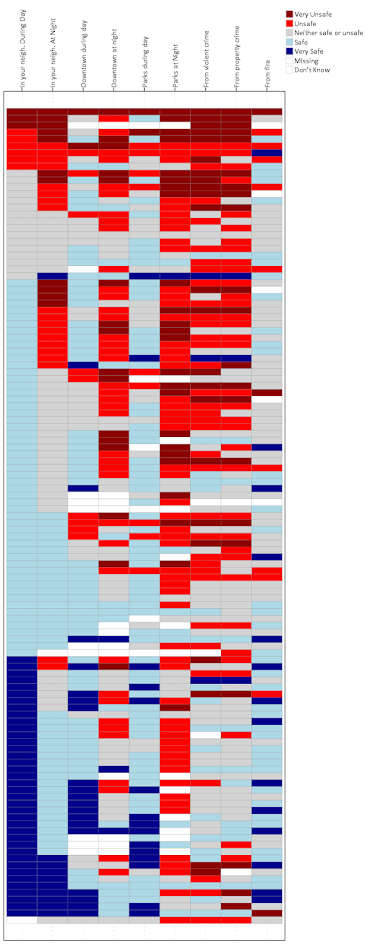So to start, normally I would use the chart builder dialog to make the skeleton for the GGRAPH code and update that. Here if you make a scatterplot in the chart dialog and assign the color it gets you most of the way there. But I will walk through some of the other steps.

• `TEMPORARY.` and then `SELECT IF` – these two steps are to only draw a heatmap for survey responses for the around 100 individuals from council district 1. Subsequently the `EXECUTE.` command at the end makes it so the `TEMPORARY` command is over.
• Then for in the inline GPL code, `PAGE: begin(scale(800px,2000px))` changes the chart dimensions to taller and skinnier than the default chart size in SPSS. Also note you need a corresponding `PAGE: end()` command when you use a `PAGE: begin()` command.
• `GUIDE: axis(dim(1), opposite())` draws the labels for the X axis on the top of the graph, instead of the bottom.
• `GUIDE: axis(dim(2), null())` prevents drawing the Y axis, which just uses the survey id to displace survey responses
• `SCALE: cat(aesthetic` maps different colors to each different survey response. Feeling safe are given blues, and not safe are given red colors. I gave neutral grey and missing white as well.
• `SCALE: cat(dim(2), sort.data(), reverse())`, this tells SPSS to draw the Y axis in the order in which the data are already sorted. Because I sorted the Q6 variables before I did the VARSTOCASES this sorts the responses with the most fear to the top.
• The `ELEMENT: polygon(` statement just draws the squares, and then specifies to color the interior of the squares according to the Q6 variable. I given the outline of the squares a grey color, but white works nice as well. (Black is a bit overpowering.)

So now you have the idea. But like I said this can be hard to identify overall patterns sometimes. So sometimes I like to limit the responses in the graph. Here I make a heatmap of the full dataset (over 1,500 responses), but just look at the different types of missing data. Red is system missing in the original dataset, and Black is the survey filled in "Don’t Know".

``````*Missing data representation.
TEMPORARY.
SELECT IF (Q6 = 9 OR Q6 = 8).
GGRAPH
/GRAPHDATASET NAME="graphdataset" VARIABLES=QType ID Q6 MISSING = VARIABLEWISE
/GRAPHSPEC SOURCE=INLINE.
BEGIN GPL
PAGE: begin(scale(800px,2000px))
SOURCE: s=userSource(id("graphdataset"))
DATA: QType=col(source(s), name("QType"), unit.category())
DATA: ID=col(source(s), name("ID"), unit.category())
DATA: Q6=col(source(s), name("Q6"), unit.category())
GUIDE: axis(dim(1), opposite())
GUIDE: axis(dim(2), null())
SCALE: cat(aesthetic(aesthetic.color.interior), map(("1", color.darkred),("2", color.red),("3", color.lightgrey),
("4", color.lightblue), ("5", color.darkblue), ("9", color.black), ("8", color.red)))
ELEMENT: polygon(position(QType*ID), color.interior(Q6), color.exterior(color.grey), transparency.exterior(transparency."0.7"))
PAGE: end()
END GPL.
EXECUTE.``````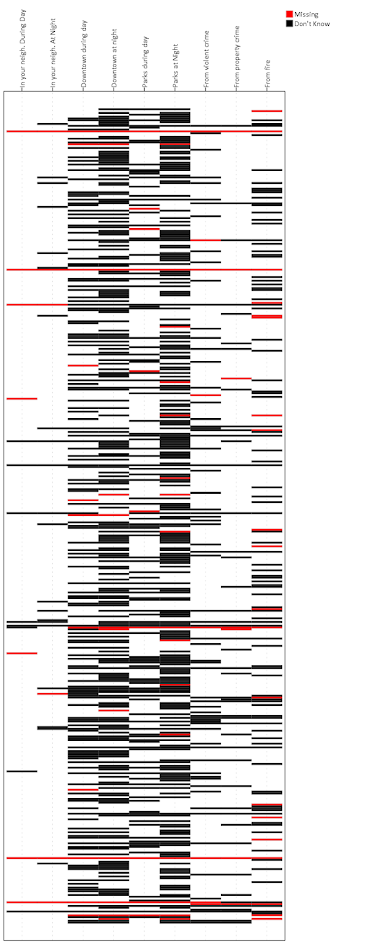You can see the system missing across all 6 questions happens very rarely, I only see three cases, but there are a ton of "Don’t Know" responses. Another way to simplify the data is to use small multiples for each type of response. Here is the first graph, but using a panel for each of the individual survey responses. See the `COORD: rect(dim(1,2), wrap())` and then the `ELEMENT` statement for the updates. As well as making the size of the chart shorter and fatter, and not drawing the legend.

``````*Small multiple.
TEMPORARY.
SELECT IF DISTRICT = 1.
GGRAPH
/GRAPHDATASET NAME="graphdataset" VARIABLES=QType ID Q6
/GRAPHSPEC SOURCE=INLINE.
BEGIN GPL
PAGE: begin(scale(1000px,1000px))
SOURCE: s=userSource(id("graphdataset"))
DATA: QType=col(source(s), name("QType"), unit.category())
DATA: ID=col(source(s), name("ID"), unit.category())
DATA: Q6=col(source(s), name("Q6"), unit.category())
COORD: rect(dim(1,2), wrap())
GUIDE: axis(dim(1), opposite())
GUIDE: axis(dim(2), null())
GUIDE: legend(aesthetic(aesthetic.color.interior), null())
SCALE: cat(aesthetic(aesthetic.color.interior), map(("1", color.darkred),("2", color.red),("3", color.lightgrey),
("4", color.lightblue), ("5", color.darkblue), ("9", color.white), ("8", color.white)))
SCALE: cat(dim(2), sort.data(), reverse())
ELEMENT: polygon(position(QType*ID*Q6), color.interior(Q6), color.exterior(color.grey), transparency.exterior(transparency."0.7"))
PAGE: end()
END GPL.
EXECUTE.``````You technically do not need to reshape the data using `VARSTOCASES` at first to make these heatmaps (there is an equivalent VARSTOCASES command within GGRAPH you could use), but this way is simpler in my opinion. (I could not figure out a way to use multiple response sets to make these non-aggregated charts, so if you can figure that out let me know!)

The idea of a heatmap can be extended to much larger grids — basically any raster graphic can be thought of as a heatmap. But for SPSS you probably do not want to make heatmaps that are very dense. The reason being SPSS always makes its charts in vector format, you cannot tell it to just make a certain chart a raster. So a very dense heatmap will take along time to render. But I like to use them in some situations as I have shown here with smaller N data in SPSS.

Also off-topic, but I may be working on a cook-book with examples for SPSS graphics. If I have not already made a blog post let me know what you would examples you would like to see!

# Spatial join points to polygons using Python and SPSS

A recent use case of mine I had around 60 million points that I wanted to assign to census block groups. ArcGIS was being problematic to simply load in the 60 million point dataset (let alone spatial join it), so I wrote some python code and will show using python and SPSS how to accomplish this.

First, a shout out to Rex Douglass and this blog post, I’ve adapted most of the python code here from that example. Also before we get started, it will be necessary to download several geospatial libraries for python. Here you need shapely, pyshp, and rtree. As a note, I have only been able to get these to install and work using the IOOS channel for Anaconda, e.g. `conda install -c ioos shapely rtree pyshp`. (I have not been able to get fiona to work.)

# The Python Part

So I will go through a quick rundown of the python code first. All of the data and code to run this yourself can be downloaded here. To start, I import all of the necessary libraries and functions.

``````import shapefile
from rtree import index
from shapely.geometry import Polygon, Point``````

The next step is to read in the polygon shapefile that we want to assign points to. Note you could swap this part out with fiona (if you can get it working!), but I just use the pyshp function `shapefile.Reader`. Note you need to change the `data` string to point to where the shapefile containing your polygons is located on your local machine.

``````#load in the shapefile of block groups
data = r'C:\Users\axw161530\Dropbox\Documents\BLOG\Point_inPoly_PythonSPSS'

In my data these are block groups for New York city, and they are projected into feet using a local projection. (As an FYI, you can open up the “prj” file for shapefiles in a plain text editor to see the projection.) Now, the shapefile object, `bg_NYC` here, has several iterables that you can access either the geometries or the records available. First we need to get those individual polygons and stuff into a list, and then convert into a Polygon object shapely can deal with.

``````bg_shapes = bg_NYC.shapes()  #get the iterable for the polygon boundary points
bg_points = [q.points for q in bg_shapes] #convert to list of geometry
polygons = [Polygon(q) for q in bg_points] #convert to a shapely Polygon``````

Next I am going to do two things. First to make a vector that matches those Polygons to a particular id, I need to read in the data attributes from the shapefile. This is accomplished via the `.records()` attribute. For US census geometries they have what is oft labeled a GEOID. In this example shapefile the GEOID ends up being in the second variable slot. The second thing I accomplish here is I build an rtree lookup. The motivation for this is, when we do a point in polygon check, it can be an expensive procedure the more polygons you have. You can first limit the number of potential polygons to check though by only checking whether a point falls within the bounding box of a polygon, and then do the more expensive operation on the actual (more complicated) boundary of the polygon.

``````#build spatial index from bounding boxes
#also has a second vector associating area IDs to numeric id
bg_records = bg_NYC.records() #bg_records is the geoid
idx = index.Index() #creating an rtree
c_id = 0
area_match = []
for a,b in zip(bg_shapes,bg_records):
area_match.append(b)
idx.insert(c_id,a.bbox,obj=b)
c_id += 1``````

Now we have all the necessary ingredients to make a function that inputs one X,Y point, and then returns a GEOID. First, the function turns the input X,Y points into a Point object shapely can work with. Second, it does the bounding box lookup I mentioned earlier, using the `idx` rtree that is available in the global environment. Third, it loops over those resulting polygons that intersected the bounding box, and checks to see if the point is within that polygon using the shapely operation `point.within(polygon)`. If that is true, it returns the associated GEOID, and if none are found it returns None. Again, the objects in this function `idx`, `polygons`, and `area_match` are taken from the global environment. A few additional notes: it will return the first point in polygon found, so if you have overlapping polygons this will simply return the first, not necessarily all of them. That is not the case with our census polygons here though. Second, the functionality here is for a point on the exact border between two polygons to return False.

``````#now can define function with polygons, area_match, and idx as globals
def assign_area(x,y):
point = Point(x,y)
for i in idx.intersection((x,y,x,y)):
if point.within(polygons[i]):
return area_match[i]
return None
#note points on the borders will return None``````

To test this function I have a set of points in New York for this particular projection already associated with a GEOID.

``````#now testing
test_vec = [(1003610, 239685, '360050063002'),
(1006787, 240666, '360050183022'),
( 993580, 219484, '360610122001'),
( 986385, 214971, '360610115001'),
( 947148, 167688, '360850201001'),
(      0,      0, 'Miss')]

for a,b,c in test_vec:
print [assign_area(x=a,y=b),c]``````

And this should subsequently print out at your console:

``````['360050063002', '360050063002']
['360050183022', '360050183022']
['360610122001', '360610122001']
['360610115001', '360610115001']
['360850201001', '360850201001']
[None, 'Miss']``````

For those wishing to do this in vectorized in python, check out the GeoPanda’s functionality. But here I let it churn out one by one by using SPSS.

# The SPSS Part

So once the above function is defined in your SPSS environment, we can simply use `SPSSINC TRANS` to assign XY data to a block group. Here is a quick example. First we read in some data, this is the homicide data from the New York times discussed here. It has the points projected in the same feet as the polygons were.

``````*Conducting point in polygon tests with Python and SPSS.
FILE HANDLE data /NAME = "C:\Users\axw161530\Dropbox\Documents\BLOG\Point_inPoly_PythonSPSS".
*Read in the NYC homicide data.
GET TRANSLATE FILE='data\HomPoints_JoinBG.dbf' /TYPE=DBF /MAP .
DATASET NAME HomData.``````

Now I am going to use the SPSS command `SHOW` to display the current date and time, (so you can see how long the operation takes). This dataset has 4,021 cases of homicide, and the set of polygons we are matching to has around 6,500 block groups. The time the operation takes depends on both, but the rtree search should make the number of polygons not as big a deal as simply looping through all of them. Second, I use SPSSINC TRANS to call the python function we previously constructed. Third, this dataset already has the GEOID matched to the points (via ArcGIS), so I check to make sure I get the same results as ArcGIS. In this example there are quite a few points that ArcGIS failed to return a match for, but this operation does. (It would take more investigation on my part though as to why that is the case.)

``````*Use this to show timing.
SHOW \$VAR.

*Now using SPSSINC TRANS to assign geoid.
SPSSINC TRANS RESULT=GeoID2 TYPE=12
/FORMULA "assign_area(x=XFt,y=YFt)".

SHOW \$VARS.
*Check that the operations are all correct (as compared to ArcGIS)
COMPUTE Check = (GEOID = GEOID2).
FREQ Check.``````

This example runs almost instantly. For some tests with my bigger dataset of 60 million, matching half a million points to this set of polygons took around 12 minutes.

# To End

Again, all of the data and code to run this at once can be downloaded here. I will need to make a blog post at some point of using pyproj to project point data in SPSS as well, such as to go to and from Lat-Lon to a local projection. You probably always want to do geometric operations like this and buffers with projected data, but you may get the data in Lat-Lon or want to export data in Lat-Lon to use online maps.

For those working with crime data, I oft complain that crime is frequently on the borders of census geographies. But due to slight differences in resolution, most GIS systems will still assign crime points to census geographies. I’m not sure if it is a big problem for much analysis in our field, but the proportion on the border is clearly quite large in some instances. For things that can occur often outdoors, like robberies and field stops, the proportion is even higher because crime is often recorded at intersections (I have estimates for the percentage of crimes at intersections for 14 years in Albany in this paper). So the problem depends on the crime type or nature of the incident (traffic stops are almost always listed at intersections), but I have seen analysis I would bet over 50% of the incidents are on the border of census blocks and/or block groups.

A general way to check this in GIS is to turn your polygon data into lines, and then assign points to the nearest line and check the distance. You will see many points that are very close to the border (say within 5 meters) that really should be undetermined.

I had a prior blog post on working with American Community Survey data in SPSS. The meta-data format has changed from that example though, and the Census gives out comma separated files and xls Templates now. So this will be an update, and I have good stuff for those working strictly in python, as well as those wanting to load the data is SPSS.

``````import urllib, os

downFold = r'C:\Users\axw161530\Dropbox\Documents\BLOG\ACS_Python_SPSS\Data'
base = r'http://www2.census.gov/programs-surveys/acs/summary_file/2014/data/5_year_seq_by_state/NewYork/Tracts_Block_Groups_Only/'

for i in range(1,5):  #change range(1,5) to range(1,122) to download all zip files
file = "20145ny0" + str(i).zfill(3) + "000.zip"
urllib.urlretrieve(base + file, os.path.join(downFold,file))

urllib.urlretrieve(base + "g20145ny.csv", os.path.join(downFold,"g20145ny.csv"))``````

The `downFold` string is where the files will be downloaded to (so change that to a place on your local machine), and the `base` string ends up being the base URL for that particular set of files. The files go from 1 to 121 in that example, but just to keep the time down I only download tables one through four. The second `urlib.urlretrieve` line downloads the geography csv file (we won’t be using the other geography file, which is the same data but in tab delimited format).

Now we can go and download the meta data excel file shells. For this dataset they are located here. Here we want the 5 year templates. Once that data is downloaded, then unzip all of the files. You could technically do this in python as well, but I just use 7zip, as that has a handy dialog to unzip multiple files to the same place.

So the way the files work, there are a set of estimate and margin of error text files that are comma delimited that have the demographic characteristics. (Here for all block groups and census tracts in New York.) The xls excel files contain the variable names, along with a brief description for the variables.

If you are a hipster and only do data analysis in python, here is a function that takes the location to a xls template file and the corresponding data file and reads it into a pandas data frame.

``````#this reads in american community survey data
import xlrd
import pandas as pd

book = xlrd.open_workbook(Template) #first open the xls workbook
sh = book.sheet_by_index(0)
vars = [i.value for i in sh.row(0)] #names on the first row
labs = [i.value for i in sh.row(1)] #labels on the second
#this rewrites duplicate 'BLANK' names, mangle dups not working for me
n = 0
vars2 = []
for i in range(len(vars)):
if vars[i] == 'BLANK':
n += 1
vars2.append('BLANK.' + str(n))
else:
vars2.append(vars[i])
#check for if geo file or data file
if vars2 == 'FILETYPE':
else:
return df,zip(vars2,labs)``````

In a nutshell, it reads the metadata column names and labels from the excel spreadsheet, then reads in the csv file with the data. It returns two objects, the one on the left is a pandas dataframe, and the one on the right is a zipped up list of the variable names and the variable labels. This would be a bit simpler, except that the format for the geo dataset is a little different than all the data files and contains multiple “BLANK” fields (the `mangle_dupe_cols` option in `read_csv` is not behaving like I expect it to). For the non-geographic file, I need to tell python the filetype column is a string, else it interprets the “e” in the estimate files as a scientific number (e.g. 1e5 = 100,000).

So here is an example of using this function to grab the second table. When I unzipped the excel templates, it nested the data templates in another subfolder, hence the `TemplateFold` string.

``````TemplateFold = downFold + r'\seq'
Tab002,Meta002 = readACS(TemplateFold + r'\Seq2.xls',downFold + r'\e20145ny0002000.txt')``````

If you want to check out all the variable labels, you can then do:

``````for i in Meta002:
print i ``````

Or if you want to turn that into a dictionary you can simply do `dict(Meta002)`. If you wanted to import all 121 tables and merge them you should be able to figure that out in a simple loop from here (note the “x.zfill(n)” function to pad the integers with leading zeroes). But I typically only work with a select few tables and variables at a time, so I won’t worry about that here.

The function works the same with the geographic data and its template. (Which that metadata template is not nested in the further down `seq` folder.)

``GeoDat,MetaGeo = readACS(downFold + r'\2014_SFGeoFileTemplate.xls',downFold + r'\g20145ny.csv')``

Note if you are working with both the estimates and the margin of error files, you may want to put somewhere in the code to change the variable names to signify that, such as by putting a suffix of “e” or “m”. If you just work with the estimates though you don’t need to worry about that.

# Reading ACS data into SPSS

For those working in SPSS, I’ve shown previously how to turn python data into SPSS data. I’ve started working on a function to make this simpler with pandas dataframes, but I will hold off on that for now (need to figure out datetimes and NaN’s). So what I did here was grab the meta-data from the template xls file (same as before), but from that build the necessary `DATA LIST` command in SPSS, and then just submit the command. SPSS has the added benefit of having native meta-data fields, so I can also add in the variable labels. Also, this only uses the xlrd library, in case you do not have access to pandas. (I point SPSS to Anaconda, instead of worrying about using pip with the native SPSS python install.)

So in SPSS, you would first define this function

``````*This just builds the necessary SPSS program to read in the american community survey data.
BEGIN PROGRAM Python.
#creating my own function to read in data
import xlrd, spss
def OpenACS(Template,Data):
book = xlrd.open_workbook(Template)
sh = book.sheet_by_index(0)
vars = [i.value for i in sh.row(0)]
labs = [i.value for i in sh.row(1)]
#this rewrites duplicate 'BLANK' names, mangle dups not working for me
n = 0
vars2 = []
for i in range(len(vars)):
if vars[i] == 'BLANK':
n += 1
vars2.append('BLANK.' + str(n))
else:
vars2.append(vars[i])
#check for if geo file or data file
if vars2 == 'FILETYPE':  #regular data
ncols = sh.ncols - 6 #need the end of the number of variables
ext =  ' (' + str(ncols) + 'F7.0)'
v1 = ' /FILEID FILETYPE (2A6) STUSAB (A2) CHARITER (A3) SEQUENCE (A4) LOGRECNO (A7) '
v2 = '\n '.join(vars2[6:])
Tab = Data[-10:-7] #Names the dataset based on the table number
else: #geo data file
ncols = sh.ncols
ext =  ' (' + str(ncols) + 'A255)' #255 should be big enough to fit whatever str
v1 = " / "
v2 = '\n '.join(vars2)
Tab = "Geo"
#this sets errors off, implicit missing data for block groups
spss.Submit("PRESERVE.")
spss.Submit("SET RESULTS OFF ERRORS OFF.")
#now creating the import program to read in the data
begin = "DATA LIST LIST(',') FILE = '%s'" % (Data)
full_str = begin + v1 + v2 + ext + "\n."
spss.Submit(full_str)
#assigning a dataset name
datName = "DATASET NAME Table" + Tab + "."
spss.Submit(datName)
#now adding in the variable labels
for i,j in zip(vars2,labs):
#replaces double quotes with single quotes in label
strVal = """VARIABLE LABELS %s "%s".""" % (i,j.replace('"',"'"))
spss.Submit(strVal)
if Tab == "Geo":
spss.Submit("ALTER TYPE ALL (A = AMIN).")
spss.Submit("RESTORE.")
END PROGRAM.``````

Again this is much shorter if I only needed to worry about the data files and not the geo file, but that slight formatting difference is a bit of a pain. Here I use the errors off trick to suppress the data list errors for missing data (which is intended, as not all of the data is available at the block group level). But you will still get error messages in the SPSS syntax bottom section. They can be ignored if it is the “insufficient data” warning.

Here is an example of using this python function now to read the data into SPSS. This automatically assigns a dataset name, either based on the Table number, or “Geo” for the geographic data.

``````*Now reading in the data files I want.
BEGIN PROGRAM Python.
downFold = r'C:\Users\axw161530\Dropbox\Documents\BLOG\ACS_Python_SPSS\Data'
TemplateFold = downFold + r'\seq'

#reading in Data file, table number 2
OpenACS(TemplateFold + r'\Seq2.xls',downFold + r'\e20145ny0002000.txt')

OpenACS(downFold + r'\2014_SFGeoFileTemplate.xls',downFold + r'\g20145ny.csv')
END PROGRAM.
EXECUTE.``````

And Voila, there is your small area American Community Survey data in SPSS. This will produce two different datasets, “Table002” and “TableGeo” that can be merged together via `MATCH FILES`.

Let me know in the comments if you have already worked out a function to turn pandas dataframes into SPSS datasets.

I’ve written quite a few blog posts over the years, and it is getting to be hard for me to keep all of them orderly. So I recently created a page with a simple table of contents with code snippets broken down into categories. There are also a few extra SPSS macros in there that I have not written blog posts about.

Every now and then I see a broken link and update it, but I know there are more I am missing. So just send an email if I have a link to some of my code and it is currently broken.

# Plotting panel data with many lines in SPSS

A quick blog post – so you all are not continually assaulted by my mug shot on the front page of the blog!

Panel data is complicated. When conducting univariate time series analysis, pretty much everyone plots the series. I presume people do not do this often for panel data because the charts tend to be more messy and less informative. But by using transparency and small multiple plots are easy places to start to unpack the information. Here I am going to show these using plots of arrest rates from 1970 through 2014 in New York state counties. The data and code can be downloaded here, and that zip file contains info. on where the original data came from. It is all publicly available – but mashing up the historical census data for the population counts by county is a bit of a pain.

So I will start with grabbing my created dataset, and then making a default plot of all the lines. Y axis is the arrest rate per 1,000 population, and the X axis are years.

``````*Grab the dataset.
FILE HANDLE data /NAME = "!!Your File Handle Here!!!".
GET FILE = "data\Arrest_WPop.sav".
DATASET NAME ArrestRates.

*Small multiple lines over time - default plot.
GGRAPH
/GRAPHDATASET NAME="graphdataset" VARIABLES=Year Total_Rate County
/GRAPHSPEC SOURCE=INLINE.
BEGIN GPL
SOURCE: s=userSource(id("graphdataset"))
DATA: Year=col(source(s), name("Year"))
DATA: Total_Rate=col(source(s), name("Total_Rate"))
DATA: County=col(source(s), name("County"), unit.category())
GUIDE: axis(dim(1), label("Year"))
GUIDE: axis(dim(2), label("Total Arrest Rate per 1,000"))
ELEMENT: line(position(Year*Total_Rate), split(County))
END GPL.``````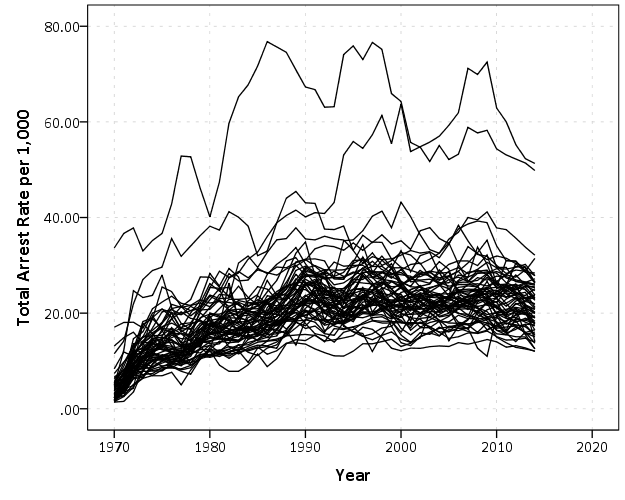That is not too bad, but we can do slightly better by making the lines small and semi-transparent (which is the same advice for dense scatterplots):

``````*Make them transparent and smaller.
FORMATS Total_Rate (F2.0).
GGRAPH
/GRAPHDATASET NAME="graphdataset" VARIABLES=Year Total_Rate County
/GRAPHSPEC SOURCE=INLINE.
BEGIN GPL
SOURCE: s=userSource(id("graphdataset"))
DATA: Year=col(source(s), name("Year"))
DATA: Total_Rate=col(source(s), name("Total_Rate"))
DATA: County=col(source(s), name("County"), unit.category())
GUIDE: axis(dim(1), label("Year"))
GUIDE: axis(dim(2), label("Total Arrest Rate per 1,000"))
SCALE: linear(dim(1), min(1970), max(2014))
ELEMENT: line(position(Year*Total_Rate), split(County), transparency(transparency."0.7"), size(size."0.7"))
END GPL.``````This helps disentangle the many lines bunched up. There appear to be two outliers, and basically the rest of the pack.

A quick way to check out each individual line is then to make small multiples. Here I wrap the panels, and make the plot size bigger. I also make the X and Y axis null. This is ok though, as I am just focusing on the shape of the trend, not the absolute level.

``````*Small multiples.
GGRAPH
/GRAPHDATASET NAME="graphdataset" VARIABLES=Year Total_Rate County
/GRAPHSPEC SOURCE=INLINE.
BEGIN GPL
PAGE: begin(scale(1000px,1000px))
SOURCE: s=userSource(id("graphdataset"))
DATA: Year=col(source(s), name("Year"))
DATA: Total_Rate=col(source(s), name("Total_Rate"))
DATA: County=col(source(s), name("County"), unit.category())
COORD: rect(dim(1,2), wrap())
GUIDE: axis(dim(1), null())
GUIDE: axis(dim(2), null())
GUIDE: axis(dim(3), opposite())
SCALE: linear(dim(1), min(1970), max(2014))
ELEMENT: line(position(Year*Total_Rate*County))
PAGE: end()
END GPL.
*Manually edited to make less space between panels.``````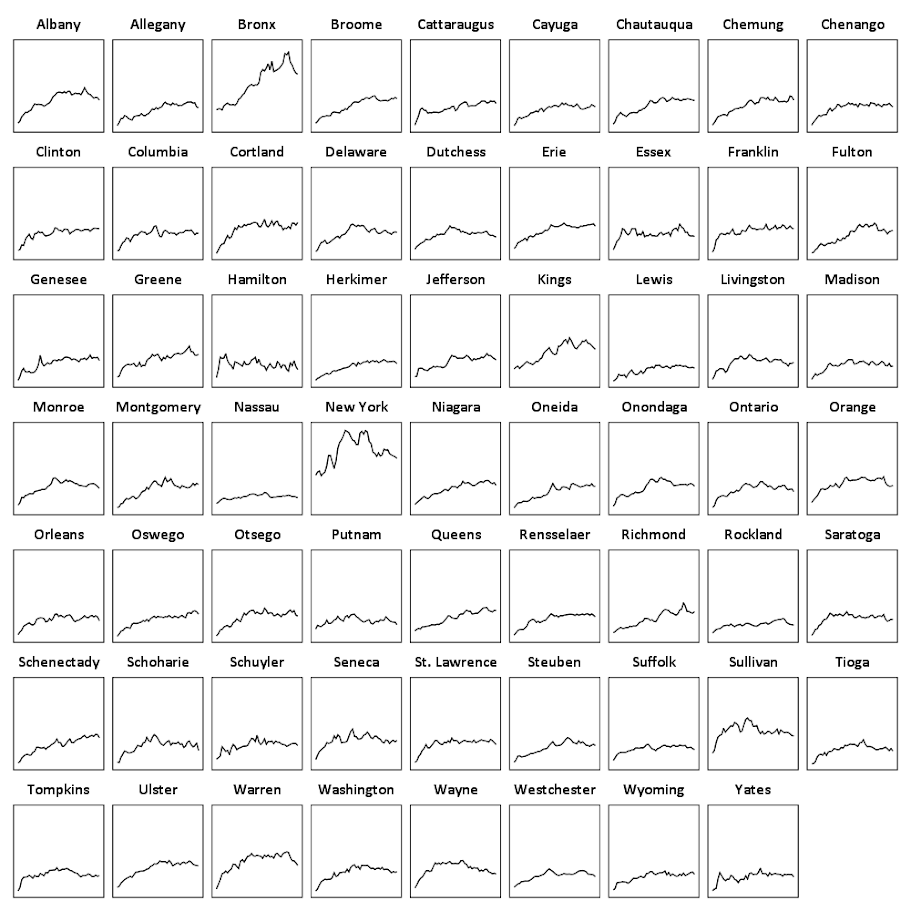There are a total of 62 counties in New York, so this is feasible. With panel sets of many more lines, you can either split the small multiple into more graphs, or cluster the lines based on the overall shape of the trend into different panels.

Here you can see that the outliers are New York county (Manhattan) and Bronx county. Bronx is a pretty straight upward trend (which mirrors many other counties), but Manhattan’s trajectory is pretty unique and has a higher variance than most other places in the state. Also you can see Sullivan county has quite a high rate compared to most other upstate counties (upstate is New York talk for everything not in New York City). But it leveled off fairly early in the time series.

This dataset also has arrest rates broken down by different categories; felony (drug, violent, dwi, other), and misdemeanor (drug, dwi, property, other). It is interesting to see that arrest rates have been increasing in most places over this long time period, even though crime rates have been going down since the 1990’s. They all appear to be pretty correlated, but let me know if you use this dataset to do some more digging. (It appears index crime totals can be found going back to 1990 here.)

# Comparing samples post-matching – some helper functions after FUZZY (SPSS)

I’ve been conducting quite a few case-control or propensity score matching studies lately. So I wrote some helper functions for use after the SPSS `FUZZY` command. These create the case-control dataset, plus calculate some of the standardized bias metrics for matching on continuous outcomes.

The use case here is if you have a sub-set of treated individuals, and you want to draw a comparison sample matched on certain characteristics (which can include just one propensity score and/or multiple covariates). Here is the macro to follow along, and I will provide a quick walkthrough of how it works. (There is documentation in the header for what the parameters are and what the function returns.)

So first I am going to import my macro using `INSERT`:

``````*Inserting the macro.
INSERT FILE = "C:\Users\andrew.wheeler\Dropbox\Documents\BLOG\Matching_StandBias\PropBalance_Macro.sps".``````

Now just for illustration I am going to make a fake dataset to illustrate the utility of matching. Here I have a universe of 2,000 people. There is a subset of treated individuals (165), but they are only selected if they are under 28 years old and male.

``````*Create a fake dataset.
SET SEED 10.
INPUT PROGRAM.
LOOP Id = 1 TO 2000.
END CASE.
END LOOP.
END FILE.
END INPUT PROGRAM.
DATASET NAME OrigData.
COMPUTE Male = RV.BERNOULLI(0.7).
COMPUTE YearsOld = RV.UNIFORM(18,40).
FORMATS Male (F1.0) YearsOld (F2.0).
DO IF Male = 1 AND YearsOld <= 28.
COMPUTE Treated = RV.BERNOULLI(0.3).
ELSE.
COMPUTE Treated = 0.
END IF.
COMPUTE #OutLogit = 0.7 + 0.5*Male - 0.05*YearsOld - 0.7*Treated.
COMPUTE #OutProb = 1/(1 + EXP(-#OutLogit)).
COMPUTE Outcome = RV.BERNOULLI(#OutProb).
FREQ Treated Outcome.``````

So what happens when we make comparisons among the entire sample, which includes females and older people?

``````*Compare means with the original full sample.
T-TEST GROUPS=Treated(0 1) /VARIABLES=Outcome.``````

We get basically no difference, our treated mean is 0.40 and the untreated mean is 0.39. But instead of comparing the 165 to the entire sample, we draw more reasonable control cases. Here we do an exact match on `Male`, and then we do a fuzzy match on `YearsOld` to within 3 years.

``````*Draw the comparison sample based on Male (exact) and YearsOld (Fuzzy).
FUZZY BY=Male YearsOld SUPPLIERID=Id NEWDEMANDERIDVARS=Match1 GROUP=Treated
EXACTPRIORITY=FALSE FUZZ=0 3 MATCHGROUPVAR=MGroup DRAWPOOLSIZE=CheckSize
/OPTIONS SAMPLEWITHREPLACEMENT=FALSE MINIMIZEMEMORY=TRUE SHUFFLE=TRUE SEED=10.``````

Now what the `FUZZY` command does in SPSS is creates a new variable, named `Match1` here, that places the matched Id in the same row as the original treated sample. You cannot easily make the updated comparisons that you want though in this data format. So after writing the code to do this about 7 times, I decided to make it into a simple macro. Here is an example of calling my macro, `!MatchedSample`.

``````*Now run my macro to make the matched sample.
!MatchedSample Dataset=OrigData Id=Id Case=Treated MatchGroup=MGroup Controls=[Match1]
MatchVars=[YearsOld] OthVars=Outcome Male.``````

This then spits out two new datasets, as well as appends a new variable to the original dataset named `MatchedSample` to show what cases have been matched. Then it is simple to see the difference in our means among our matched sample.

``````*Now the t-test with the matched sample subset.
DATASET ACTIVATE MatchedSamples.
T-TEST GROUPS=Treated(0 1) /VARIABLES=Outcome.``````

Which shows the same mean for treated, 0.40 (since all the treated were matched), but the comparison group now has a mean of 0.51, so here the treatment reduced the outcome.

The macro also provides an additional dataset named `AggStats` that estimates the standardized bias in the original sample vs. the standardized bias in the matched sample. (Standardized bias is just Cohen’s D measure multiplied by 100.) This then also calculates the standardized bias reduction for each continuous covariate. Before I forget, a neat way to test for balance jointly (instead of one variable at a time) is to conduct an additional regression equation predicting treatment and then testing for all coefficients equal to zero.

In this fake example the propensity scores would not be needed, you could just estimate a typical logistic regression equation controlling for `YearsOld` and `Male`. But the utility of matching comes from when you don’t know the functional form of how those covariates affect the outcome. So if the outcome was a very non-linear function of age, you don’t have to worry about estimating that function, you can just match on age and still get a reasonable comparison of the mean difference for treated vs. not-treated.

# Weekly and monthly graphs for monitoring crime patterns (SPSS)

I was recently asked for some code to show how I created the charts in my paper, Tables and Graphs for Monitoring Crime Patterns (Pre-print can be seen here).

I cannot share the data used in the paper, but I can replicate them with some other public data. I will use calls for service publicly available from Burlington, VT to illustrate them.

The idea behind these time-series charts are not for forecasting, but to identify anomalous patterns – such as recent spikes in the data. (So they are more in line with the ideas behind control charts.) Often even in big jurisdictions, one prolific offender can cause a spike in crimes over a week or a month. They are also good to check more general trends as well, to see if crimes have had more slight, but longer term trends up or down.

For a preview, we will be making a weekly time series chart: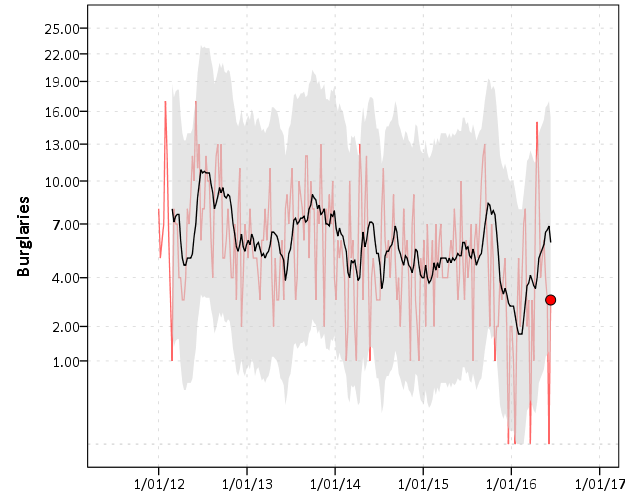In the weekly chart the red line is the actual data, the black line is the average of the prior 8 weeks, and the grey band is a Poisson confidence interval around that prior moving average. The red dot is the most recent week.

And we will also be making a monthly seasonal chart: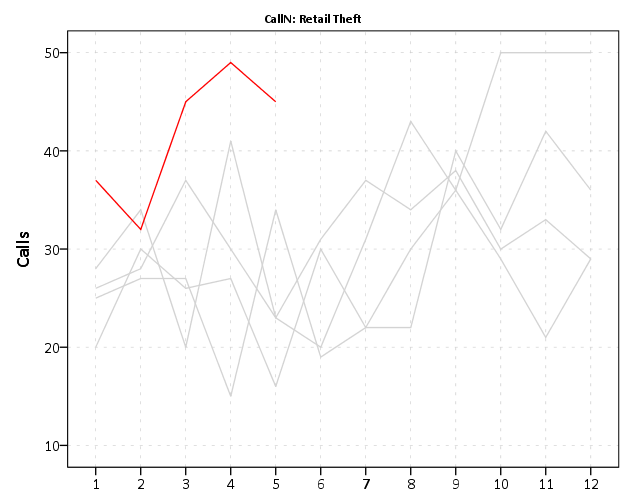The red line is the counts of calls per month in the current year, and the lighter grey lines are prior years (here going back to 2012).

So to start, I saved the 2012 through currently 6/20/2016 calls for service data as a csv file. And here is the code to read in that incident level data.

``````*Change this to where the csv file is located on your machine.
FILE HANDLE data /NAME = "C:\Users\andrew.wheeler\Dropbox\Documents\BLOG\Tables_Graphs".
GET DATA  /TYPE=TXT
/FILE="data\Calls_for_Service_Dashboard_data.csv"
/ENCODING='UTF8'
/DELCASE=LINE
/DELIMITERS=","
/QUALIFIER='"'
/ARRANGEMENT=DELIMITED
/FIRSTCASE=2
/DATATYPEMIN PERCENTAGE=95.0
/VARIABLES=
AlcoholRelated AUTO
Area AUTO
CallDateTime AUTO
CallType AUTO
Domv AUTO
DayofWeek AUTO
DrugRelated AUTO
EndDateTime AUTO
GeneralTimeofDay AUTO
IncidentNumber AUTO
LocationType AUTO
MentalHealthRelated AUTO
MethodofEntry AUTO
Month AUTO
PointofEntry AUTO
StartDateTime AUTO
Street AUTO
Team AUTO
Year AUTO
/MAP.
CACHE.
EXECUTE.
DATASET NAME CFS.``````

First I will be making the weekly chart. What I did when I was working as an analyst was make a chart that showed the recent weekly trends and to identify if the prior week was higher than you might expect it to be. The weekly patterns can be quite volatile though, so I smoothed the data based on the average of the prior eight weeks, and calculated a confidence interval around that average count (based on the Poisson distribution).

As a start, we are going to turn our date variable, `CallDateTime`, into an SPSS date variable (it gets read in as a string, AM/PM in date-times are so annoying!). Then we are going to calculate the number of days since some baseline – here it is 1/1/2012, which is Sunday. Then we are going to calculate the weeks since that Sunday. Lastly we select out the most recent week, as it is not a full week.

``````*Days since 1/1/2012.
COMPUTE #Sp = CHAR.INDEX(CallDateTime," ").
COMPUTE Days = DATEDIFF(CallDate,DATE.MDY(1,1,2012),"DAYS").
COMPUTE Weeks = TRUNC( (Days-1)/7 ).
FREQ Weeks /FORMAT = NOTABLE /STATISTICS = MIN MAX.
SELECT IF Weeks < 233.``````

Here I do weeks since a particular date, since if you do `XDATE.WEEK` you can have not full weeks. The magic number 233 can be replaced by sometime like `SELECT IF Weeks < (\$TIME - 3*24*60*60).` if you know you will be running the syntax on a set date, such as when you do a production job. (Another way is to use `AGGREGATE` to figure out the latest date in the dataset.)

Next what I do is that when you use `AGGREGATE` in SPSS, there can be missing weeks with zeroes, which will mess up our charts. There end up being 22 different call-types in the Burlington data, so I make a base dataset (named `WeekFull`) that has all call types for each week. Then I aggregate the original calls for service dataset to CallType and Week, and then I merge the later into the former. Finally I then recode the missings intos zeroes.

``````*Make sure I have a full set in the aggregate.
FREQ CallType.
AUTORECODE CallType /INTO CallN.
*22 categories, may want to collapse a few together.
INPUT PROGRAM.
LOOP #Weeks = 0 TO 232.
LOOP #Calls = 1 TO 22.
COMPUTE CallN = #Calls.
COMPUTE Weeks = #Weeks.
END CASE.
END LOOP.
END LOOP.
END FILE.
END INPUT PROGRAM.
DATASET NAME WeekFull.

*Aggregate number of tickets to weeks.
DATASET ACTIVATE CFS.
DATASET DECLARE WeekCalls.
AGGREGATE OUTFILE='WeekCalls'
/BREAK Weeks CallN
/CallType = FIRST(CallType)
/TotCalls = N.

*Merge Into WeekFull.
DATASET ACTIVATE WeekFull.
MATCH FILES FILE = *
/FILE = 'WeekCalls'
/BY Weeks CallN.
DATASET CLOSE WeekCalls.
*Missing are zero cases.
RECODE TotCalls (SYSMIS = 0)(ELSE = COPY).``````

Now we are ready to calculate our statistics and make our charts. First we create a date variable that represents the beginning of the week (for our charts later on.) Then I use `SPLIT FILE` and `CREATE` to calculate the prior moving average only within individiual call types. The last part of the code calculates a confidence interval around prior moving average, and assumes the data is Poisson distributed. (More discussion of this is in my academic paper.)

``````DATASET ACTIVATE WeekFull.
COMPUTE WeekBeg = DATESUM(DATE.MDY(1,1,2012),(Weeks*7),"DAYS").

*Moving average of prior 8 weeks.
SORT CASES BY CallN Weeks.
SPLIT FILE BY CallN.
CREATE MovAv = PMA(TotCalls,8).
*Calculating the plus minus 3 Poisson intervals.
COMPUTE #In = (-3/2 + SQRT(MovAv)).
DO IF #In >= 0.
COMPUTE LowInt = #In**2.
ELSE.
COMPUTE LowInt = 0.
END IF.
COMPUTE HighInt = (3/2 + SQRT(MovAv))**2.
EXECUTE.``````

If you rather use the inverse of the Poisson distribution I have notes in the code at the end to do that, but they are pretty similar in my experience. You also might consider (as I mention in the paper), rounding fractional values for the `LowInt` down to zero as well.

Now we are ready to make our charts. The last data manipulation is to just put a flag in the file for the very last week (which will be marked with a large red circle). I use `EXECUTE` before the chart just to make sure the variable is available. Finally I keep the `SPLIT FILE` on, which produces 22 charts, one for each call type.

``````IF Weeks = 232 FinCount = TotCalls.
EXECUTE.

*Do a quick look over all of them.
GGRAPH
/GRAPHDATASET NAME="graphdataset" VARIABLES=WeekBeg TotCalls MovAv LowInt HighInt FinCount MISSING=VARIABLEWISE
/GRAPHSPEC SOURCE=INLINE.
BEGIN GPL
SOURCE: s=userSource(id("graphdataset"))
DATA: WeekBeg=col(source(s), name("WeekBeg"))
DATA: TotCalls=col(source(s), name("TotCalls"))
DATA: MovAv=col(source(s), name("MovAv"))
DATA: LowInt=col(source(s), name("LowInt"))
DATA: HighInt=col(source(s), name("HighInt"))
DATA: FinCount=col(source(s), name("FinCount"))
SCALE: pow(dim(2), exponent(0.5))
GUIDE: axis(dim(1))
GUIDE: axis(dim(2), label("Crime Count"))
ELEMENT: line(position(WeekBeg*TotCalls), color(color.red), transparency(transparency."0.4"))
transparency.interior(transparency."0.4"), transparency.exterior(transparency."1"))
ELEMENT: line(position(WeekBeg*MovAv))
ELEMENT: point(position(WeekBeg*FinCount), color.interior(color.red), size(size."10"))
END GPL.
SPLIT FILE OFF.``````

This is good for the analyst, I can monitor many series. Here is an example the procedure produces for mental health calls:The current value is within the confidence band, so it is not alarmingly high. But we can see that they have been trending up over the past few years. Plotting on the square root scale makes the Poisson count data have the same variance, but a nice thing about the SPLIT FILE approach is that SPSS does smart Y axis ranges for each individual call type.

You can update this to make plots for individual crimes, and here I stuff four different crime types into a small multiple plot. I use a `TEMPORARY` and `SELECT IF` statement before the `GGRAPH` code to select out the crime types I am interested in.

``````FORMATS TotCalls MovAv LowInt HighInt FinCount (F3.0).
TEMPORARY.
SELECT IF ANY(CallN,3,10,13,17).
GGRAPH
/GRAPHDATASET NAME="graphdataset" VARIABLES=WeekBeg TotCalls MovAv LowInt HighInt FinCount CallN MISSING=VARIABLEWISE
/GRAPHSPEC SOURCE=INLINE.
BEGIN GPL
PAGE: begin(scale(900px,600px))
SOURCE: s=userSource(id("graphdataset"))
DATA: WeekBeg=col(source(s), name("WeekBeg"))
DATA: TotCalls=col(source(s), name("TotCalls"))
DATA: MovAv=col(source(s), name("MovAv"))
DATA: LowInt=col(source(s), name("LowInt"))
DATA: HighInt=col(source(s), name("HighInt"))
DATA: FinCount=col(source(s), name("FinCount"))
DATA: CallN=col(source(s), name("CallN"), unit.category())
COORD: rect(dim(1,2), wrap())
SCALE: pow(dim(2), exponent(0.5))
GUIDE: axis(dim(1))
GUIDE: axis(dim(2), start(1), delta(3))
GUIDE: axis(dim(3), opposite())
GUIDE: form.line(position(*,0),color(color.lightgrey),shape(shape.half_dash))
ELEMENT: line(position(WeekBeg*TotCalls*CallN), color(color.red), transparency(transparency."0.4"))
transparency.interior(transparency."0.4"), transparency.exterior(transparency."1"))
ELEMENT: line(position(WeekBeg*MovAv*CallN))
ELEMENT: point(position(WeekBeg*FinCount*CallN), color.interior(color.red), size(size."10"))
PAGE: end()
END GPL.
EXECUTE.``````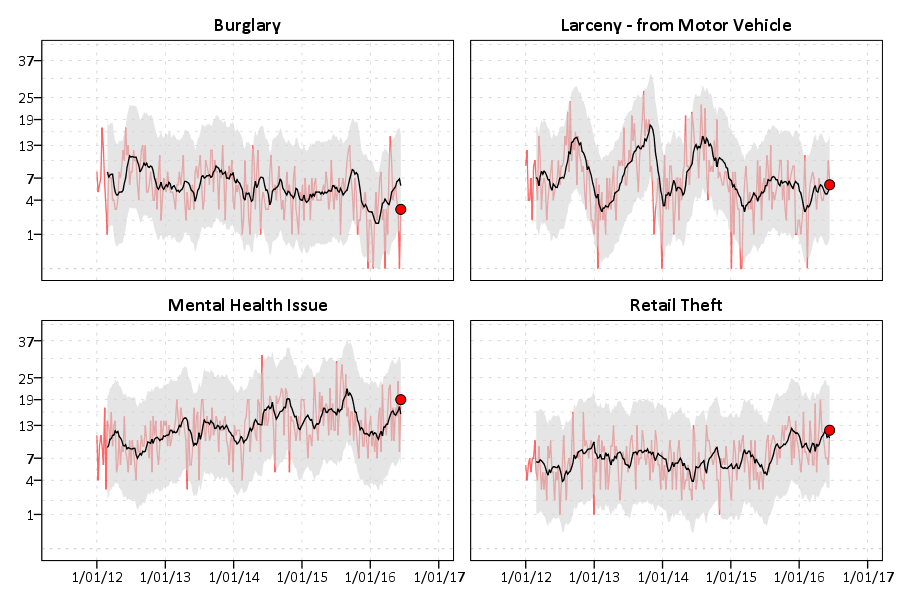You could do more fancy time-series models to create the confidence bands or identify the outliers, (exponential smoothing would be similar to just the prior moving average I show) but this ad-hoc approach worked well in my case. (I wanted to make more fancy models, but I did not let the perfect be the enemy of the good to get at least this done when I was employed as a crime analyst.)

Now we can move onto making our monthly chart. These weekly charts are sometimes hard to visualize with highly seasonal data. So what this chart does is gives each year a new line. Instead of drawing error bars, the past years data show the typical variation. It is then easy to see seasonal ups-and-downs, as well as if the latest month is an outlier.

Getting back to the code — I activate the original calls for service database and then close the Weekly database. Then it is much the same as for weeks, but here I just use calendar months and match to a full expanded set of calls types and months over the period. (I do not care about normalizing months, it is ok that February is only 28 days).

``````DATASET ACTIVATE CFS.
DATASET CLOSE WeekFull.

COMPUTE Month = XDATE.MONTH(CallDate).
COMPUTE Year = XDATE.YEAR(CallDate).

DATASET DECLARE AggMonth.
AGGREGATE OUTFILE = 'AggMonth'
/BREAK Year Month CallN
/MonthCalls = N.

INPUT PROGRAM.
LOOP #y = 2012 TO 2016.
LOOP #m = 1 TO 12.
LOOP #call = 1 TO 22.
COMPUTE CallN = #call.
COMPUTE Year = #y.
COMPUTE Month = #m.
END CASE.
END LOOP.
END LOOP.
END LOOP.
END FILE.
END INPUT PROGRAM.
DATASET NAME MonthAll.

MATCH FILES FILE = *
/FILE = 'AggMonth'
/BY Year Month CallN.
DATASET CLOSE AggMonth.``````

Next I select out the most recent month of the date (June 2016) since it is not a full month. (When I originally made these charts I would normalize to days and extrapolate out for my monthly meeting. These forecasts were terrible though, even only extrapolating two weeks, so I stopped doing them.) Then I calculate a variable called `Current` – this will flag the most recent year to be red in the chart.

``````COMPUTE MoYr = DATE.MDY(Month,1,Year).
FORMATS MoYr (MOYR6) Year (F4.0) Month (F2.0).
SELECT IF MoYr < DATE.MDY(6,1,2016).
RECODE MonthCalls (SYSMIS = 0)(ELSE = COPY).

*Making current year red.
COMPUTE Current = (Year = 2016).
FORMATS Current (F1.0).

SORT CASES BY CallN MoYr.
SPLIT FILE BY CallN.

*Same thing with the split file.
GGRAPH
/GRAPHDATASET NAME="graphdataset" VARIABLES=Month MonthCalls Current Year
/GRAPHSPEC SOURCE=INLINE.
BEGIN GPL
SOURCE: s=userSource(id("graphdataset"))
DATA: Month=col(source(s), name("Month"), unit.category())
DATA: MonthCalls=col(source(s), name("MonthCalls"))
DATA: Current=col(source(s), name("Current"), unit.category())
DATA: Year=col(source(s), name("Year"), unit.category())
GUIDE: axis(dim(1))
GUIDE: axis(dim(2), label("Calls"), start(0))
GUIDE: legend(aesthetic(aesthetic.color.interior), null())
SCALE: cat(aesthetic(aesthetic.color.interior), map(("0",color.lightgrey),("1",color.red)))
ELEMENT: line(position(Month*MonthCalls), color.interior(Current), split(Year))
END GPL.``````

You can again customize this to be individual charts for particular crimes or small multiples. You can see in the example at the beginning of the post Retail thefts are high for March, April and May. I was interested to examine overdoses, as the northeast (and many parts of the US) are having a problem with heroin at the moment. In the weekly charts they are so low of counts it is hard to see any trends though.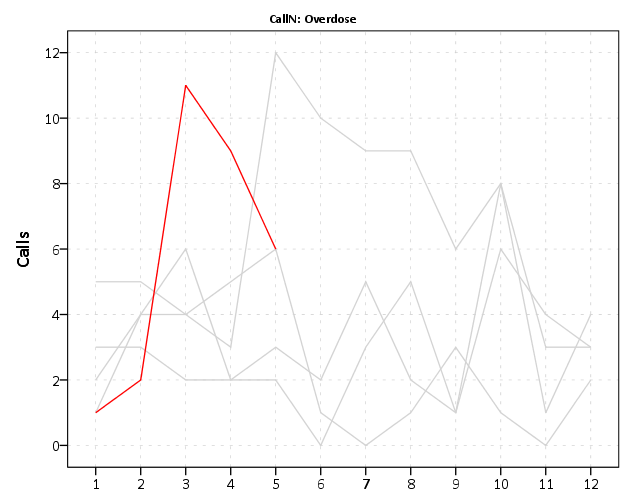We can see that overdoses were high in March. The other highest line are months in 2015, so it looks like a problem here in Burlington, but it started around a year ago.

For low counts of crime (say under 20 per month) seasonality tends to be hard to spot. For crimes more frequent though you can often see pits and peaks in summer and winter. It is not universal that crimes increase in the summer though. For ordinance violations (and ditto for Noise complaints) we can see a pretty clear peak in September. (I don’t know why that is, there is likely some logical explanation for it though.)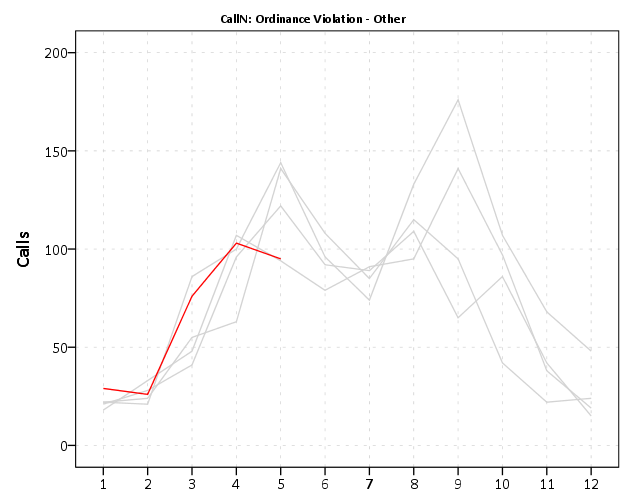My main motivation to promote these is to replace terrible CompStat tables of year-over-year percent changes. All of these patterns I’ve shown are near impossible to tell from tables of counts per month.

Finally if you want to export your images to place into another report, you can use:

``OUTPUT EXPORT /PNG IMAGEROOT = "data\TimeGraphs.png".``

PNG please – simple vector graphics like these should definately not be exported as jpegs.

Here is a link to the full set of syntax and the csv data to follow along. I submitted to doing an hour long training session at the upcoming IACA conference on this, so hopefully that gets funded and I can go into this some more.

# Shape, color, and pattern constants in SPSS charts

I have a version of the SPSS (Statistics) Version 24 GPL reference guide bookmarked here. The reference guide is great to skim through and see what is possible in SPSS charts – especially the set of examples on pages 329 to 411.

On page 413 they also give a set of constant colors, shapes, and texture patterns you can use in charts. Colors you can also specify in RGB scale, but it is often convenient to just say color.red or color.pink etc. Shapes and patterns for practical purposes you have to choose among the constants. (Technically in the chart template you can edit the cycle styles, and change a circle to an ellipse for example, or change the points for a dash pattern, but this would be painful for anything besides a few constants.)

Here is a handy reference guide to actually visualize those constants. Many you can guess what they look like, but the colors are more subtle. Who knew there were differences between tomato, salmon, and pink! (The tomato is more like tomato soup color.)

Here are the color constants (you can open the chart in a new tab to see a larger image):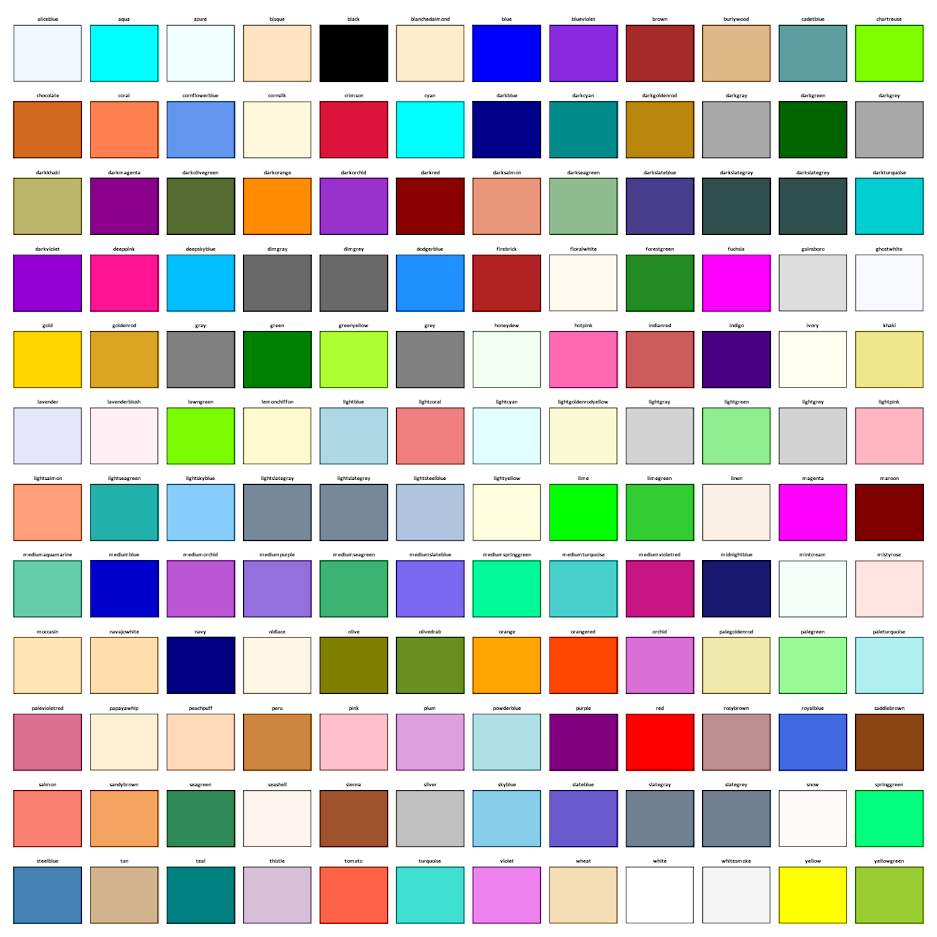The shape constants: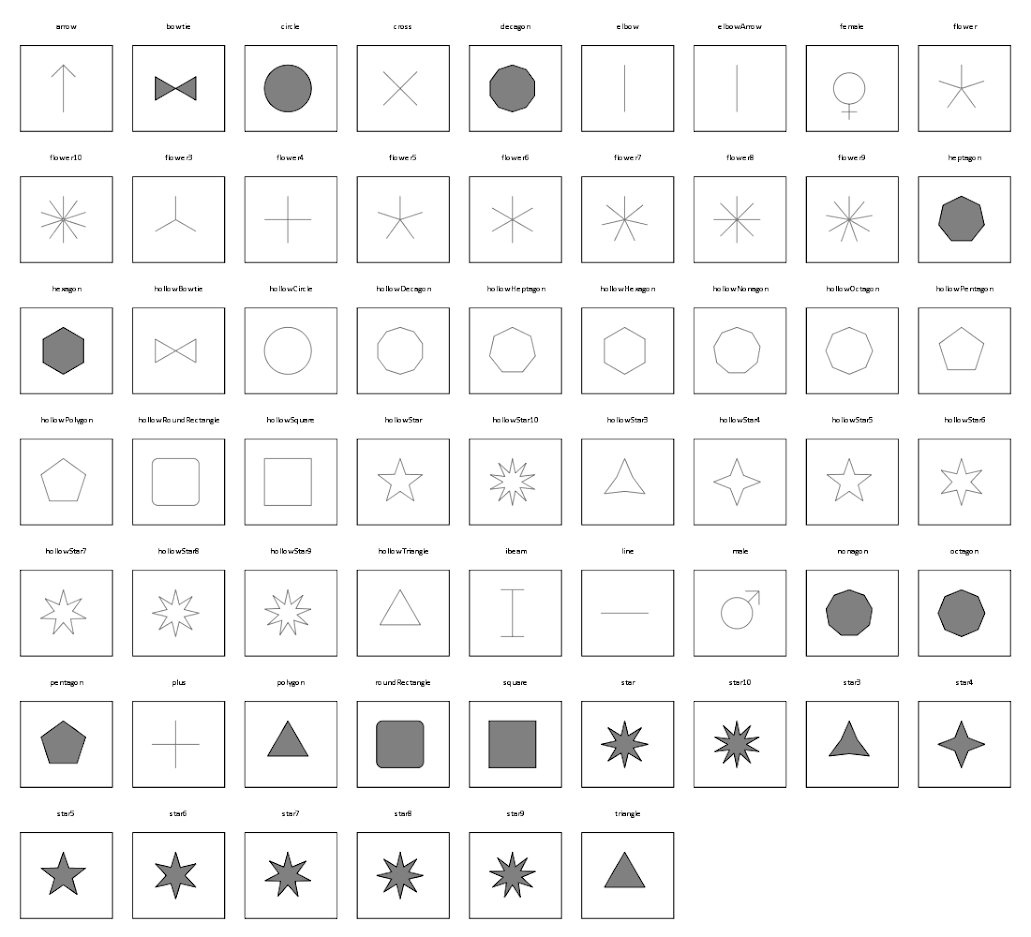The elbow and the elbowArrow do not look correct – but will take some more time to investigate. The others look ok to me though. (The number of sides and star points appear to me to be something you can also manipulate in the chart template cycles, if for some reason you want a hendecagon).

And here are the pattern constants. I plot them with a grey filled interior – you can see some specifically only have an outline and always have a transparent fill: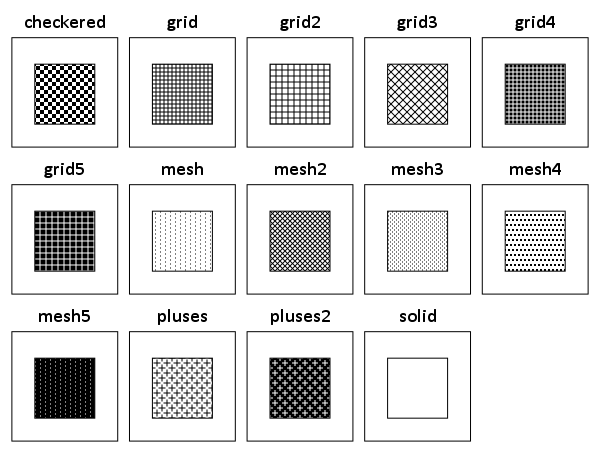Here is code to replicate the charts, and here is a PDF to download with the constants. The colors and shapes are hard to read because they are squeezed in so small, but you can zoom into the PDF and read the categories. I haven’t used dashed lines ever, so I omit those constants here. (Patterns I use pretty rarely, but I have used them if there are only two categories.)

A useful change for the colors would be sorting in a logical order. They are just currently in alphabetical. I am too lazy though to convert the colors to a colorspace and sort them though. (Maybe converting the PDF to SVG would do the trick easy enough though.)

# Maps in inline GPL statements (SPSS)

Here I will go through an example of using inline GPL statements to import map backgrounds in SPSS charts. Here you can download the data and code to follow along with this post. This is different than using maps via VIZTEMPLATE, as I will show.

Note you can also use the graphboard template chooser to make some default maps, but I’ve never really learned how to make them on my own. For example, say I want to map that sets both the color and the transparency of areas based on different attributes. This is not possible with the current selection of map templates that comes with SPSS (V22).

But I figured out some undocumented ways to import maps into inline GPL code, and you can get pretty far with just the possibilities available within the grammar of graphics.

The data I will be using is a regular grid of values across DC. What I calculated was the hour of the day with the most Robberies over along time period (2011 through 2015 data) using a weighted average approach synonymous with geographically weighted regression. Don’t take this too seriously though, as there appears to be some errors in the time fields for the historical DC crime data.

So below I first define a handle to where my data is stored, recode the hour field into a smaller set of bins, and then make a scatterplot.

``````FILE HANDLE data /NAME = "C:\Users\andrew.wheeler\Dropbox\Documents\BLOG\Inline_Maps_GGRAPH".

GET FILE = "data\MaxRobHour.sav".
DATASET NAME MaxRob.

*Basic Scatterplot.
FREQ HourEv.
RECODE HourEv (0 THRU 3 = 1)(11 THRU 19 = 2)(ELSE = COPY) INTO HourBin.
VALUE LABELS HourBin
1 '0 to 3'
2 '11 to 19'.

DATASET ACTIVATE MaxRob.
* Chart Builder.
GGRAPH
/GRAPHDATASET NAME="graphdataset" VARIABLES=XMetFish[LEVEL=SCALE] YMetFish[LEVEL=SCALE] HourBin
MISSING=LISTWISE REPORTMISSING=NO
/GRAPHSPEC SOURCE=INLINE.
BEGIN GPL
SOURCE: s=userSource(id("graphdataset"))
DATA: XMetFish=col(source(s), name("XMetFish"))
DATA: YMetFish=col(source(s), name("YMetFish"))
DATA: HourBin=col(source(s), name("HourBin"), unit.category())
GUIDE: axis(dim(1), label("XMetFish"))
GUIDE: axis(dim(2), label("YMetFish"))
GUIDE: legend(aesthetic(aesthetic.color.exterior), label("HourBin"))
ELEMENT: point(position(XMetFish*YMetFish), color.exterior(HourBin))
END GPL.``````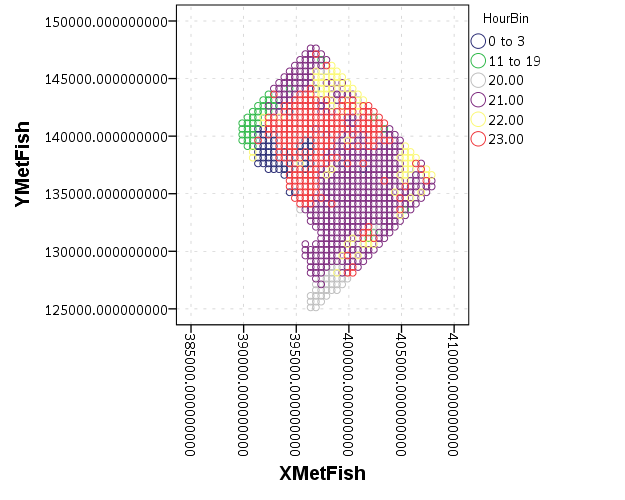We can do quite a bit to make this map look nicer. Here I change:

• make the aspect ratio 1 to 1, and set the map limits
• get rid of the X and Y axis (the particular projected coordinates make no difference)
• make a nice set of colors based on a ColorBrewer palatte and map the color to the interior of the point

And below that is the map it produces.

``````*Making chart nice, same aspect ratio, colors, drop x & y.
FORMATS HourBin (F2.0).
GGRAPH
/GRAPHDATASET NAME="graphdataset" VARIABLES=XMetFish[LEVEL=SCALE] YMetFish[LEVEL=SCALE] HourBin
MISSING=LISTWISE REPORTMISSING=NO
/GRAPHSPEC SOURCE=INLINE.
BEGIN GPL
SOURCE: s=userSource(id("graphdataset"))
DATA: XMetFish=col(source(s), name("XMetFish"))
DATA: YMetFish=col(source(s), name("YMetFish"))
DATA: HourBin=col(source(s), name("HourBin"), unit.category())
COORD: rect(dim(1,2), sameRatio())
GUIDE: axis(dim(1), null())
GUIDE: axis(dim(2), null())
GUIDE: legend(aesthetic(aesthetic.color.exterior), label("HourBin"))
SCALE: linear(dim(1), min(389800), max(408000))
SCALE: linear(dim(2), min(125000), max(147800))
SCALE: cat(aesthetic(aesthetic.color.interior), map(("1",color."810f7c"),("2",color."edf8fb"),("20",color."bfd3e6"),("21",color."9ebcda"),
("22",color."8c96c6"),("23",color."8856a7")))
ELEMENT: point(position(XMetFish*YMetFish), color.interior(HourBin))
END GPL.``````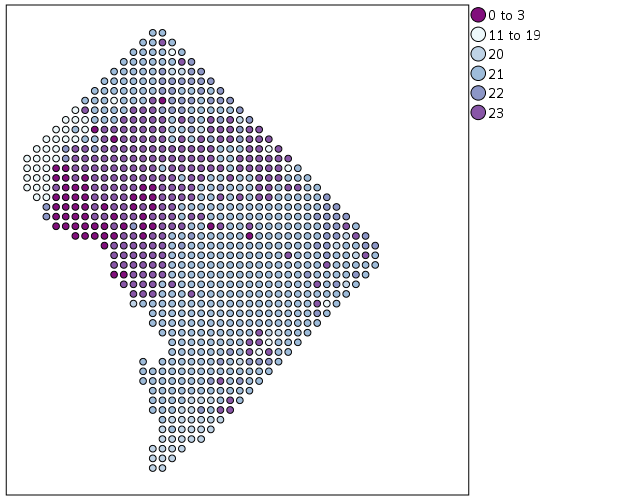So that is not too shabby a map for just plain SPSS. Now it is a bit hard to vizualize the patterns though, because the surface has needless discontinuities because of the circles. We can use squares as the shape and just do some experimentation to figure out the size needed to fill up each grid cell. Also pro-tip when making choropleth maps, with many areas often light outlines look nicer than black ones.

``````*Alittle nicer, squares, no outline.
GGRAPH
/GRAPHDATASET NAME="graphdataset" VARIABLES=XMetFish[LEVEL=SCALE] YMetFish[LEVEL=SCALE] HourBin
MISSING=LISTWISE REPORTMISSING=NO
/GRAPHSPEC SOURCE=INLINE.
BEGIN GPL
SOURCE: s=userSource(id("graphdataset"))
DATA: XMetFish=col(source(s), name("XMetFish"))
DATA: YMetFish=col(source(s), name("YMetFish"))
DATA: HourBin=col(source(s), name("HourBin"), unit.category())
COORD: rect(dim(1,2), sameRatio())
GUIDE: axis(dim(1), null())
GUIDE: axis(dim(2), null())
GUIDE: legend(aesthetic(aesthetic.color.exterior), label("HourBin"))
SCALE: linear(dim(1), min(389800), max(408000))
SCALE: linear(dim(2), min(125000), max(147800))
SCALE: cat(aesthetic(aesthetic.color.interior), map(("1",color."810f7c"),("2",color."edf8fb"),("20",color."bfd3e6"),("21",color."9ebcda"),
("22",color."8c96c6"),("23",color."8856a7")))
ELEMENT: point(position(XMetFish*YMetFish), color.interior(HourBin), shape(shape.square), size(size."9.5"),
transparency.exterior(transparency."1"))
END GPL.``````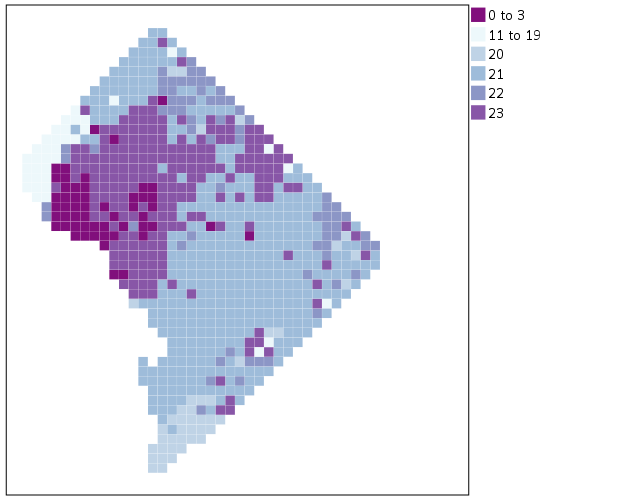Again looking pretty good for just a map in plain SPSS. With the larger squares it is easier to clump together areas with similar patterns for the peak robbery time. The city never sleeps in Georgetown it appears. A few of the polygons though are very hard to see on the edge of DC though, so we will add in the outline. See the `SOURCE: mapsrc`, `DATA: lon*lat`, and the `ELEMENT: polygon` lines for how this is done. The “DCOutline.smz” is the map template file created by SPSS.

``````*Now include the outline.
GGRAPH
/GRAPHDATASET NAME="graphdataset" VARIABLES=XMetFish[LEVEL=SCALE] YMetFish[LEVEL=SCALE] HourBin
MISSING=LISTWISE REPORTMISSING=NO
/GRAPHSPEC SOURCE=INLINE.
BEGIN GPL
SOURCE: s=userSource(id("graphdataset"))
DATA: XMetFish=col(source(s), name("XMetFish"))
DATA: YMetFish=col(source(s), name("YMetFish"))
DATA: HourBin=col(source(s), name("HourBin"), unit.category())
SOURCE: mapsrc = mapSource(file("C:\\Users\\andrew.wheeler\\Dropbox\\Documents\\BLOG\\Inline_Maps_GGRAPH\\DCOutline.smz"))
DATA: lon*lat = mapVariables(source(mapsrc))
COORD: rect(dim(1,2), sameRatio())
GUIDE: axis(dim(1), null())
GUIDE: axis(dim(2), null())
GUIDE: legend(aesthetic(aesthetic.color.exterior), label("HourBin"))
SCALE: linear(dim(1), min(389800), max(408000))
SCALE: linear(dim(2), min(125000), max(147800))
SCALE: cat(aesthetic(aesthetic.color.interior), map(("1",color."810f7c"),("2",color."edf8fb"),("20",color."bfd3e6"),("21",color."9ebcda"),
("22",color."8c96c6"),("23",color."8856a7")))
ELEMENT: point(position(XMetFish*YMetFish), color.interior(HourBin), shape(shape.square), size(size."9.5"),
transparency.exterior(transparency."1"))
ELEMENT: polygon(position(lon*lat))
END GPL.``````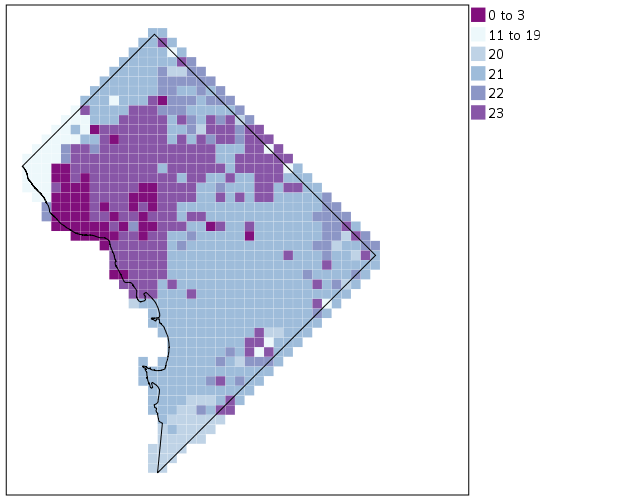Now we have a bit more of a reference. The really late at night area appears to be north of Georgetown. The reason I figured this was even possible is that although `mapSource` is not documented in the GPL reference guide, there is an example using it with the `project` function (see page 194).

Now, if I were only making one map this isn’t really much of a help – I would just export the data values, make it in ArcGIS and be done with it. But, one of the things hard to do in GIS is make small multiple maps. That is something we can do fairly easily in stat. software though. For an example, here I make a random map to compare with the observed patterns. The grammar automatically recognizes `lon*lat*Type` and replicates the background outline across each panel. Also I change the size of the overall plot using `PAGE` statements. I just typically experiment until it looks nice.

``````*Can use the outline to do small multiples.
COMPUTE HourRand = TRUNC(RV.UNIFORM(0,24)).
RECODE HourRand (0 THRU 3 = 1)(4 THRU 19 = 2)(ELSE = COPY).
VARSTOCASES
/MAKE Hour FROM HourBin HourRand
/INDEX Type.
VALUE LABELS Type 1 'Observed' 2 'Random'.

*Small multiple.
GGRAPH
/GRAPHDATASET NAME="graphdataset" VARIABLES=XMetFish YMetFish Hour Type
MISSING=LISTWISE REPORTMISSING=NO
/GRAPHSPEC SOURCE=INLINE.
BEGIN GPL
PAGE: begin(scale(1000px,500px))
SOURCE: s=userSource(id("graphdataset"))
DATA: XMetFish=col(source(s), name("XMetFish"))
DATA: YMetFish=col(source(s), name("YMetFish"))
DATA: Hour=col(source(s), name("Hour"), unit.category())
DATA: Type=col(source(s), name("Type"), unit.category())
SOURCE: mapsrc = mapSource(file("C:\\Users\\andrew.wheeler\\Dropbox\\Documents\\BLOG\\Inline_Maps_GGRAPH\\DCOutline.smz"))
DATA: lon*lat = mapVariables(source(mapsrc))
COORD: rect(dim(1,2), sameRatio(), wrap())
GUIDE: axis(dim(1), null())
GUIDE: axis(dim(2), null())
GUIDE: axis(dim(3), opposite())
GUIDE: legend(aesthetic(aesthetic.color.exterior), label("HourBin"))
SCALE: linear(dim(1), min(389800), max(408000))
SCALE: linear(dim(2), min(125000), max(147800))
SCALE: cat(aesthetic(aesthetic.color.interior), map(("1",color."810f7c"),("2",color."edf8fb"),("20",color."bfd3e6"),("21",color."9ebcda"),
("22",color."8c96c6"),("23",color."8856a7")))
ELEMENT: point(position(XMetFish*YMetFish*Type), color.interior(Hour), shape(shape.square), size(size."8"),
transparency.exterior(transparency."1"))
ELEMENT: polygon(position(lon*lat*Type))
PAGE: end()
END GPL.``````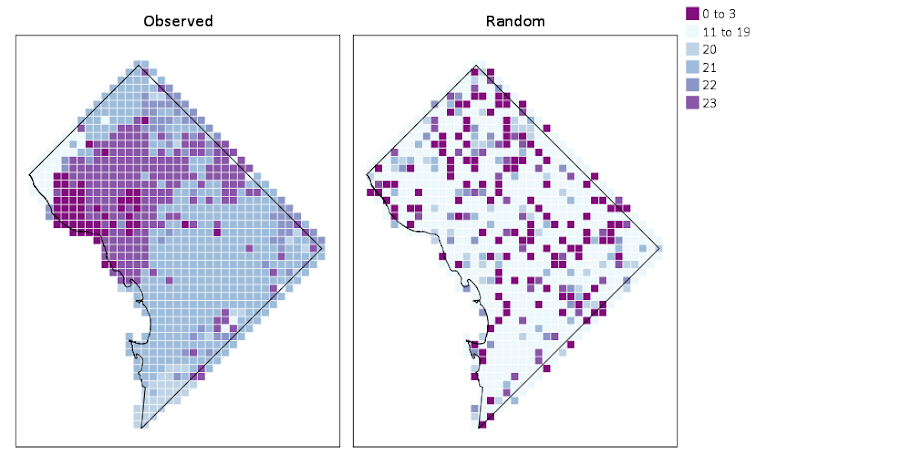We can see that this extreme amount of clustering is clearly not random.

This example works out quite nice because the micro level areas are a regular grid, so I can simulate a choropleth map look by just using square point markers. Unfortunately, I was not able to figure out how to map areas to merge a map file and an id like you can in VIZTEMPLATE. You can see some of my attempts in the attached code. You can however have multiple `mapSource` statements, so you could import say a street network, rivers and parks and map a nice background map right in SPSS. Hopefully IBM updates the documentation so I can figure out how to make a choropleth map in inline GPL statements.